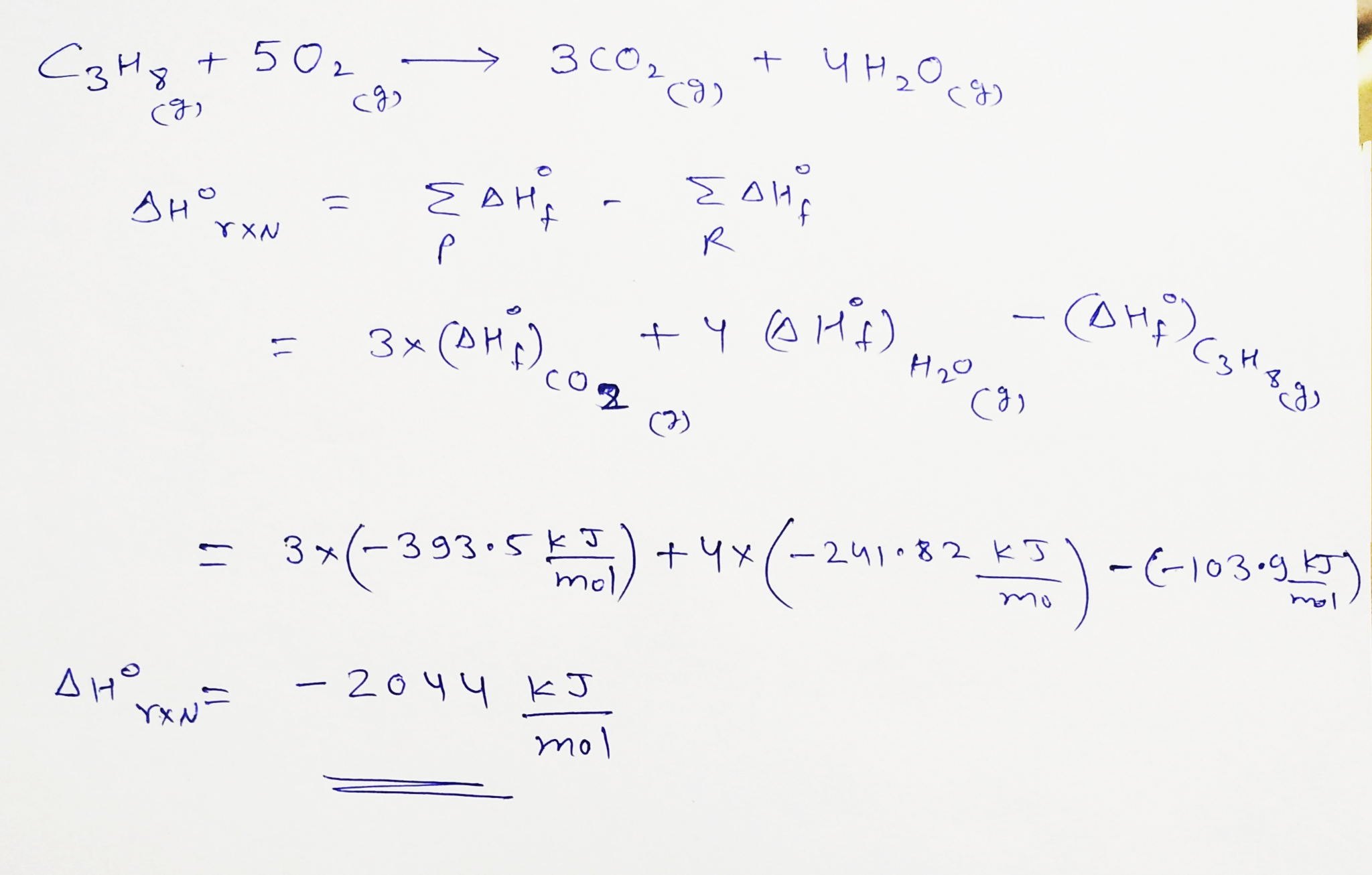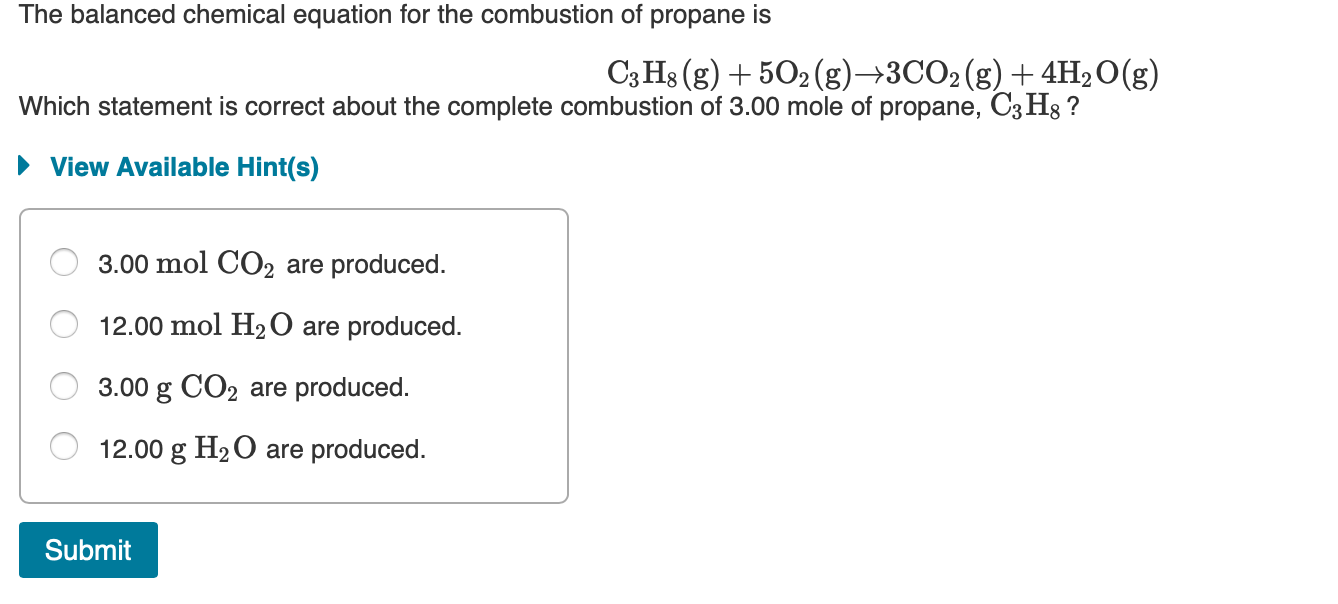Question

# ﻿Propane (C3H8)burns according to the following balanced equation: C3H8(g)+5O2(g)→3CO2(g)+4H2O(g) Calculate ΔH∘rxnΔ for this reaction using standard...

﻿Propane (C3H8)burns according to the following balanced equation:

C3H8(g)+5O2(g)→3CO2(g)+4H2O(g)

Calculate ΔH∘rxnΔ for this reaction using standard enthalpies of formation. (The standard enthalpy of formation of gaseous propane is -103.9 kJ/molkJ/mol.)

Express the enthalpy in kilojoules to four significant figures.#### Earn Coins

Coins can be redeemed for fabulous gifts.

Similar Homework Help Questions
• ### Thermochemistry

Propane burns according to the following balanced equation:C3H8 + 5O2 --> 3CO2 + 4H2OCalculate delta Hrxn for this reaction using standard enthalpies of formation. (The standard enthalpy of formation of gaseous propane is -103.9 kJ/mol.)

• ### The propane fuel (C3H8) used in gas barbeques burns according to this thermochemical equation. C3H8(g)+5O2(g)→3CO2(g)+4H2O(g)ΔH∘rxn=−2044kJ If...

The propane fuel (C3H8) used in gas barbeques burns according to this thermochemical equation. C3H8(g)+5O2(g)→3CO2(g)+4H2O(g)ΔH∘rxn=−2044kJ If a pork roast must absorb 1.7×103 kJ to fully cook, and if only 15 % of the heat produced by the barbeque is actually absorbed by the roast, what mass of CO2 is emitted into the atmosphere during the grilling of the pork roast?

• ### The propane fuel (C3H8) used in gas barbecues burns according to the following equation: C3H8(g)+5O2(g)→3CO2(g)+4H2O(g)ΔH∘rxn=−2044kJ PART...

The propane fuel (C3H8) used in gas barbecues burns according to the following equation: C3H8(g)+5O2(g)→3CO2(g)+4H2O(g)ΔH∘rxn=−2044kJ PART A: If a pork roast must absorb 2.3×103kJ to fully cook, and if only 10.% of the heat produced by the barbecue is actually absorbed by the roast, what mass of CO2 is emitted into the atmosphere during the grilling of the pork roast?

• ### The combustion of propane, C3H8, occurs via the reaction C3H8(g)+5O2(g)→3CO2(g)+4H2O(g) with heat of formation values given...

The combustion of propane, C3H8, occurs via the reaction C3H8(g)+5O2(g)→3CO2(g)+4H2O(g) with heat of formation values given by the following table: Substance ΔH∘f (kJ/mol) C3H8 (g)=  -104.7 CO2(g)= −393.5 H2O(g)= −241.8 Calculate the enthalpy for the combustion of 1 mole of propane.

• ### The propane fuel (C3H8) used in gas barbeques burns according to the following thermochemical equation: C3H8(g)+5O2(g)...

The propane fuel (C3H8) used in gas barbeques burns according to the following thermochemical equation:C3H8(g)+5O2(g)→3CO2(g)+4H2O(g) ΔH rxn= 2217kJPart AIf a pork roast must absorb 1500kJ to fully cook, and if only 12% of the heat produced by the barbeque is actually absorbed by the roast, what mass of CO2 is emitted into the atmosphere during the grilling of the pork roast?Express your answer using two significant figures. m=_____________________g

• ### Consider the combustion of propane: C3H8 (g) + 5O2 (g) → 3CO2 (g) + 4H2O(l) ΔH...

Consider the combustion of propane: C3H8 (g) + 5O2 (g) → 3CO2 (g) + 4H2O(l) ΔH = –2221 kJ Assume that all of the heat comes from the combustion of propane. Calculate ΔH in which 5.00 g of propane is burned in excess oxygen at constant pressure.

• ### The propane fuel (C3H8) used in gas barbeques burns according to the following thermochemical equation: C3H8(g)+5O2(g)...

The propane fuel (C3H8) used in gas barbeques burns according to the following thermochemical equation: C3H8(g)+5O2(g)?3CO2(g)+4H2O(g) If a pork roast must absorb 1700kJ to fully cook, and if only 14% of the heat produced by the barbeque is actually absorbed by the roast, what mass of CO2 is emitted into the atmosphere during the grilling of the pork roast? Express your answer using two significant figures.

• ### calculate the enthalpy of the combustion of propane for your barbeque C3H8 + 5O2 -> 3CO2 + 4H2O propane -105 kJ...

calculate the enthalpy of the combustion of propane for your barbeque C3H8 + 5O2 -> 3CO2 + 4H2O propane -105 kJ/mol CO2 -394 kJ/mol Water -286 kJ/mol Ooxygen. 0

• ### The balanced chemical equation for the combustion of propane is C3H8(g) + 5O2(g) +3CO2(g) + 4H2O(g)...The balanced chemical equation for the combustion of propane is C3H8(g) + 5O2(g) +3CO2(g) + 4H2O(g) Which statement is correct about the complete combustion of 3.00 mole of propane, C3Hg ? ► View Available Hint(s) O 3.00 mol CO2 are produced. O 12.00 mol H2O are produced. O 3.00 g CO2 are produced. O 12.00 g H2O are produced. Submit

• ### GIVEN INFO LP gas burns according to the following exothermic reaction: C3H8(g)+5O2(g)→3CO2(g)+4H2O(g) ΔH∘rxn= −2044kJ What mass...

GIVEN INFO LP gas burns according to the following exothermic reaction: C3H8(g)+5O2(g)→3CO2(g)+4H2O(g) ΔH∘rxn= −2044kJ What mass of LP gas is necessary to heat 1.4 L of water from room temperature (25.0 C) to boiling (100.0 C)? Assume that during heating, 16% of the heat emitted by the LP gas combustion goes to heat the water. The rest is lost as heat to the surroundings. Express your answer using two significant figures. FINAL ANWSER SHOULD BE IN GRAMS ?